# Subtraction of Fractions

Go back to  'Fractions'

 1 Introduction to Fractions 2 Steps to Subtracting Fractions 3 Subtracting Fractions with Same Denominator 4 Subtract Fractions with Different Denominators 5 Subtracting Fractions with Whole Numbers 6 Subtracting Fractions with Variables 7 Important Notes 8 Solved Examples 9 Practice Questions 10 Tips and Tricks 11 Maths Olympiad Sample Papers 12 Frequently Asked Questions (FAQs)

We at Cuemath believe that Math is a life skill. Our Math Experts focus on the “Why” behind the “What.” Students can explore from a huge range of interactive worksheets, visuals, simulations, practice tests, and more to understand a concept in depth.

Book a FREE trial class today! and experience Cuemath's LIVE Online Class with your child.

## Introduction to Fractions

Fractions are part of a whole. Before moving to the subtraction of fractions, let us revise about fractions here

## Steps to Subtracting Fractions

We have learnt to subtract whole numbers (example: $$4 -2 = 2$$).

Similarly, we can subtract fractions.

Subtracting Fractions include: 

• Subtracting fractions with whole numbers
\begin{align} \left(3\frac {1}{2} - 2 \right) \end{align}
• Subtracting fractions with like denominators
\begin{align} \left(\frac {3}{4} - \frac {1}{4} \right) \end{align}
• Subtracting fractions with different denominators
\begin{align} \left(\frac {3}{5} - \frac {1}{2} \right) \end{align}
• Subtracting  fractions with variables
\begin{align} \left(\frac {3}{5} x - \frac {1}{4}x \right) \end{align}

## Subtracting Fractions with the Same Denominator

Let us subtract the fractions \begin{align} \frac {2}{5} \end{align} and \begin{align} \frac {1}{5} \end{align} using rectangular models.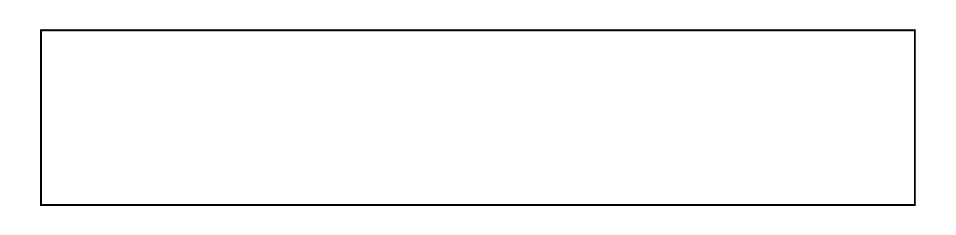We will represent \begin{align} \frac {2}{5} \end{align}  in this model by shading $$2$$ out of $$5$$ parts.

We will further shade out $$1$$ part from our shaded parts of the model to represent removing \begin{align} \frac {1}{5} \end{align}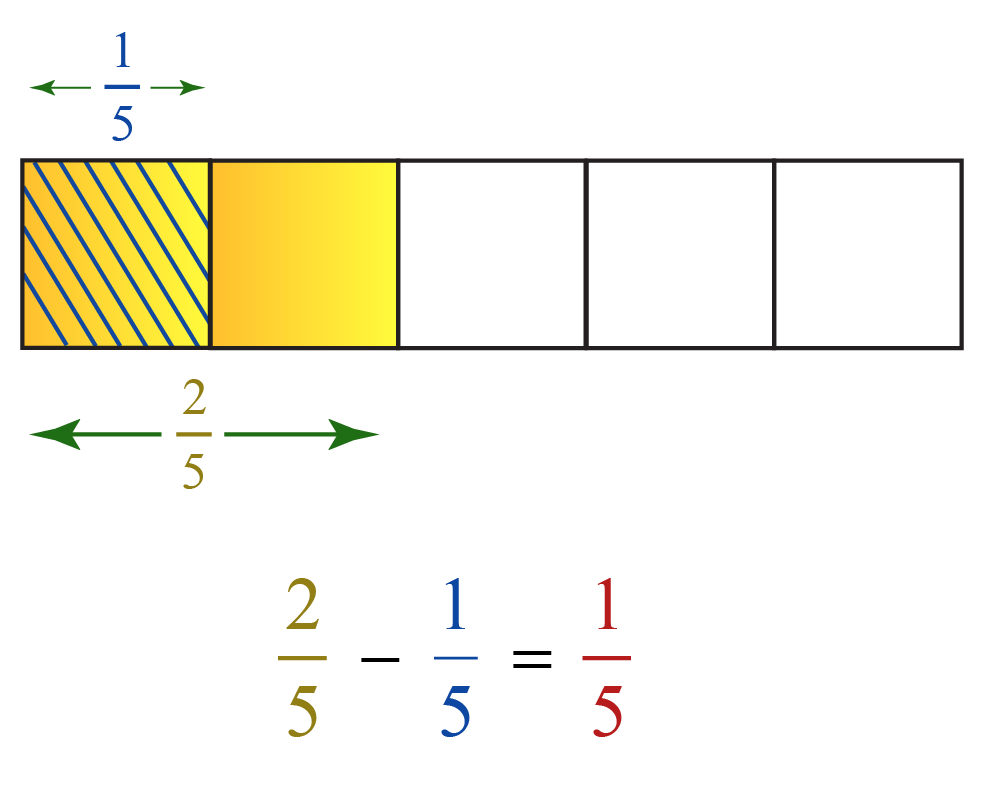We are now left with $$1$$ part in the shaded parts of the model.

Therefore,

\begin{align} \frac {2}{5} \end{align} - \begin{align} \frac {1}{5} \end{align} = \begin{align} \frac {1}{5} \end{align}

## Subtract Fractions with Different Denominators

Let us understand how to subtract unlike fractions using the area model.

\begin{align} \frac {3}{5} \end{align} - \begin{align} \frac {1}{3} \end{align}

This indicates that we have to remove \begin{align} \frac {1}{3}^{rd} \end{align} part from \begin{align} \frac {3}{5} \end{align}

Let us use our rectangle model and represent \begin{align} \frac {3}{5} \end{align} as shown.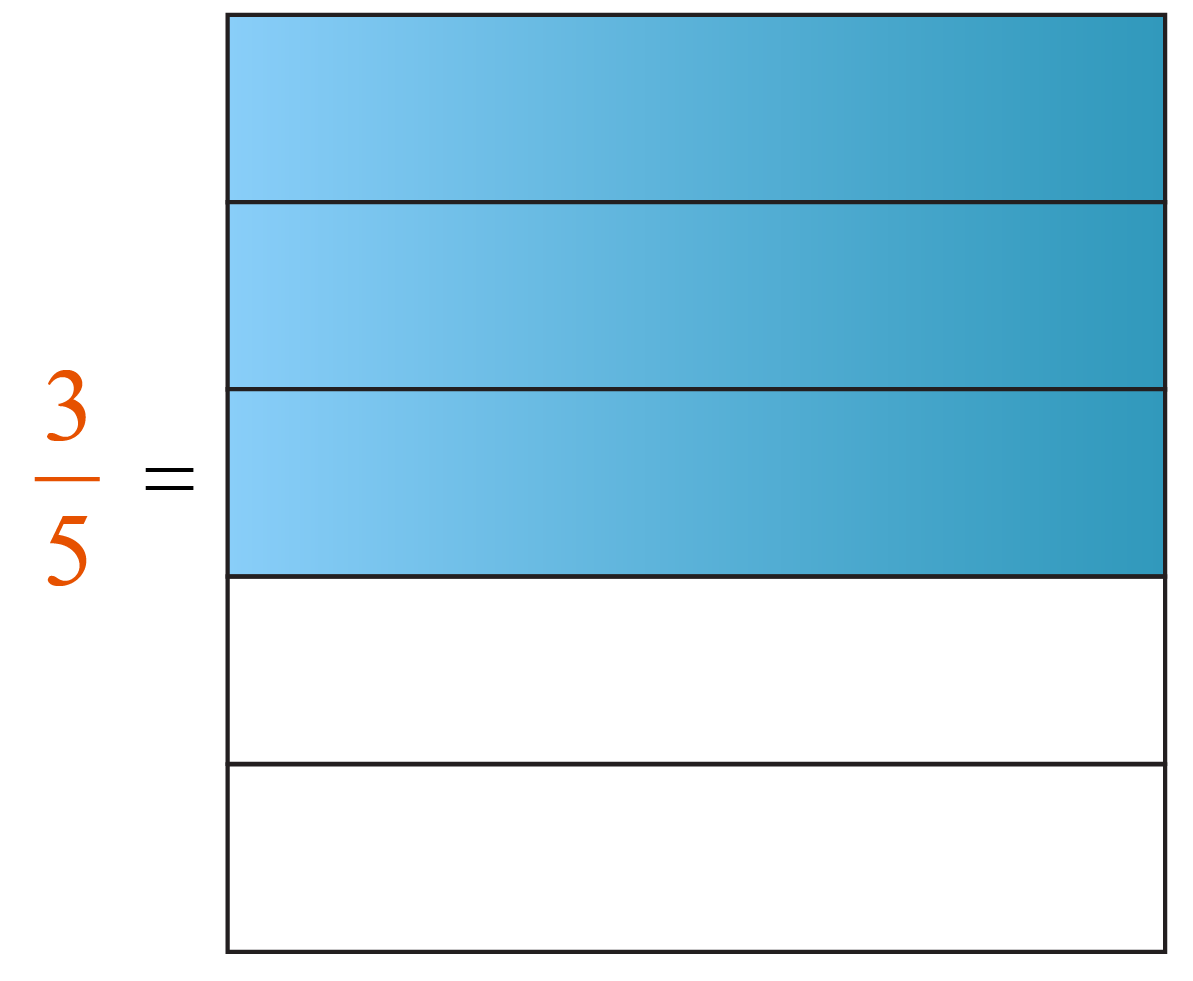\begin{align} \frac {1}{3} \end{align}  when represented vertically will have $$1$$ column shaded out of $$3$$ columns.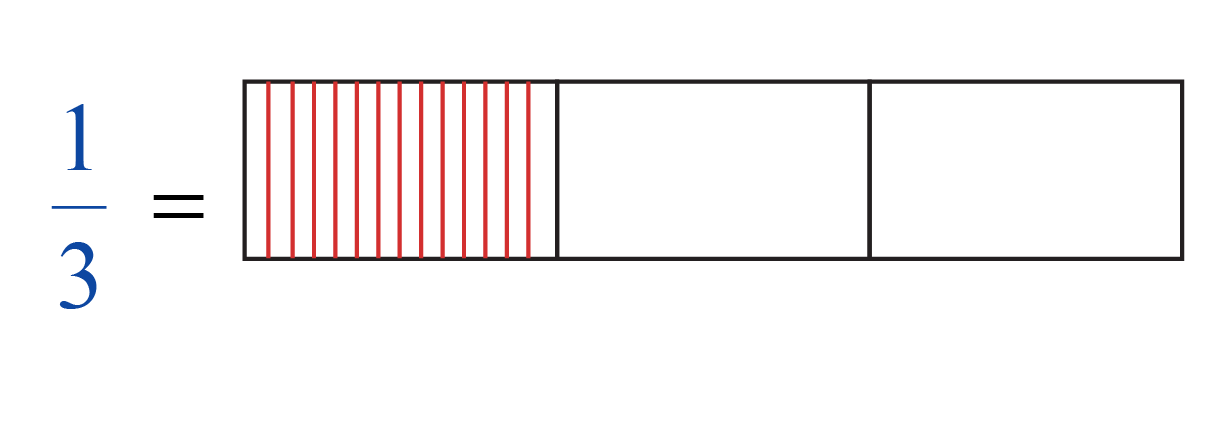Now let us represent both in the same model.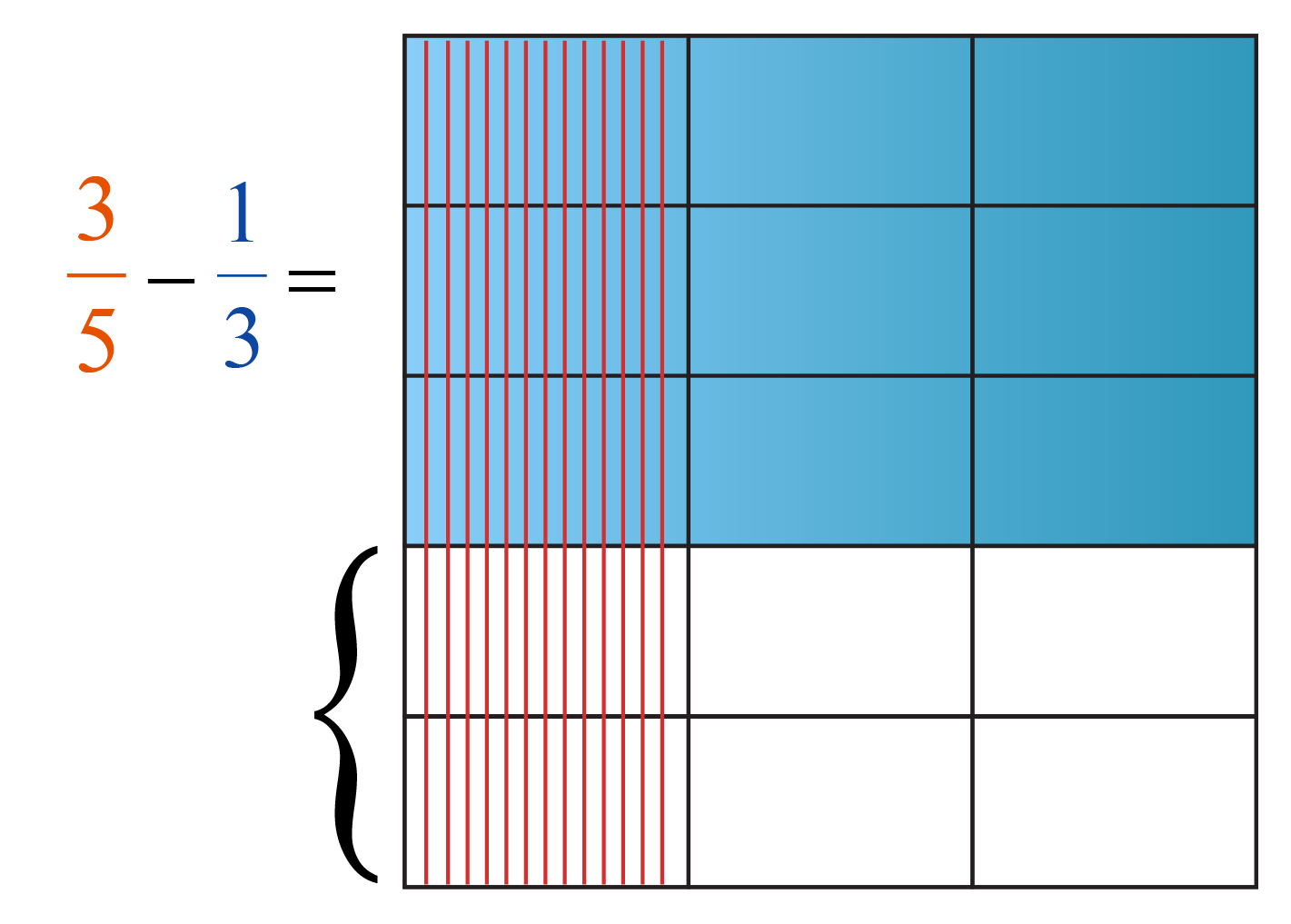We see that our model is divided into $$15$$ parts. This is our denominator.

This is nothing but the LCM of the denominators of the given fraction.

As we need to remove \begin{align} \frac {1}{3} \end{align}  from \begin{align} \frac {3}{5} \end{align}, we will move the selected two parts which are not part of the \begin{align} \frac {3}{5} \end{align}, to remove it from\begin{align} \frac {3}{5} \end{align}

Now, our model will look like this.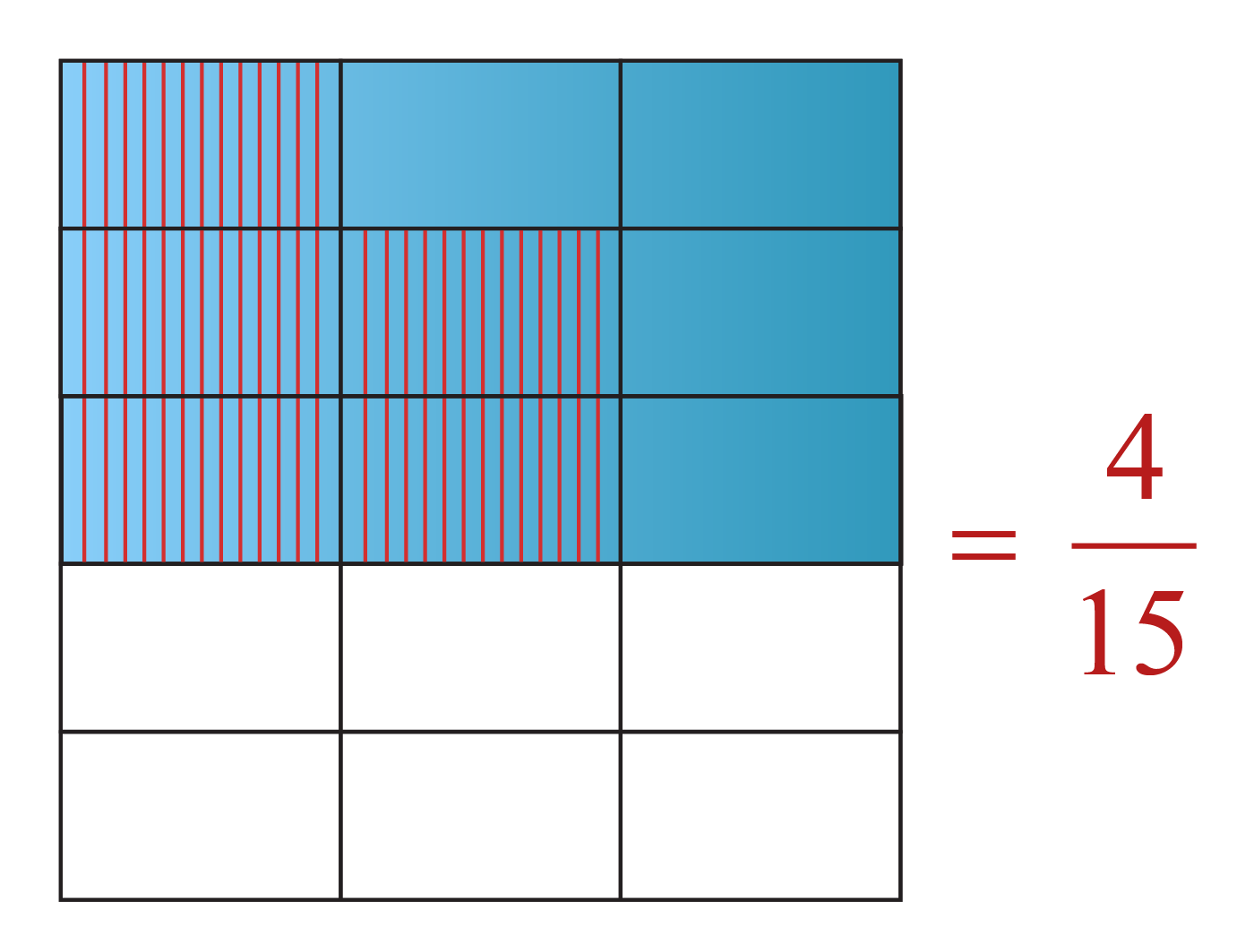We see that there are only $$4$$ parts of the remaining which are not shaded out.

Therefore,

\begin{align} \frac {3}{5} \end{align}  - \begin{align} \frac {1}{3} \end{align} = \begin{align} \frac {4}{15} \end{align}

To subtract unlike fractions,

1. Take the LCM of the denominators
2. Convert the given fractions to equivalent fractions with the denominator as the LCM
3. Subtract the numerators

## Subtracting Fractions With Whole Numbers

To subtract a fraction from a whole number, consider the following example.

\begin{align}3 - \frac {1}{2} \end{align}

Convert the whole number to a fractional form

\begin{align} 3= \frac {3}{1}\end{align}

Subtract them like unlike fraction

\begin{align} \frac {3}{1} - \frac {1}{2} \end{align}

\begin{align} &= \left(\frac {3 }{1} \times \frac {2}{2}\right) - \frac {1}{2} \\\ &= \frac {6}{2} - \frac {1}{2} \\\ &= \frac {5}{2} \\\ &= 2\frac {1}{2} \end{align}

CLUEless in Math? Check out how CUEMATH Teachers will explain Subtraction of Fractions to your kid using interactive simulations & worksheets so they never have to memorise anything in Math again!

Explore Cuemath Live, Interactive & Personalised Online Classes to make your kid a Math Expert. Book a FREE trial class today!

## Subtracting Fractions with Variables

Consider this example,  \begin{align} \frac {2}{5} x\end{align} - \begin{align} \frac {1}{5} x\end{align}

They are like terms as they have the same denominator and $$\text x$$ is common. We can take the common factor out and rewrite it as:

\begin{align} \frac {2}{5} x\end{align} - \begin{align} \frac {1}{5} x\end{align} = (\begin{align} \frac {2}{5} \end{align} - \begin{align} \frac { 1}{5} \end{align})$$x$$ =  \begin{align} \frac {1}{5} x\end{align}

Similarly, when we have to subtract \begin{align} \frac {1}{3} x\end{align} from \begin{align} \frac {1}{2} x\end{align}

We can take the LCM of the denominator and convert them to like terms and take the common variable out are rewrite it as follows:

LCM ($$2 , 3$$ = 6 )

\begin{align} \frac {1}{2} x\end{align} =  \begin{align} \frac {1}{2} x\end{align} $$\times$$  \begin{align} \frac {3}{3} \end{align} =  \begin{align} \frac {3}{6} x\end{align}

\begin{align} \frac {1}{3} x\end{align} =  \begin{align} \frac {1}{3} x\end{align} $$\times$$  \begin{align} \frac {2}{2} \end{align} =  \begin{align} \frac {2}{6} x\end{align}

\begin{align} \frac {1}{2} x - \frac {1}{3} x &= \frac {3}{6} x - \frac {2}{6} x \\ &= \left(\frac {3-2}{6} \right)x \\ &= \frac {1}{6} x\end{align}

More Important Topics
Numbers
Algebra
Geometry
Measurement
Money
Data
Trigonometry
Calculus